# Cross Multiplication Method

Cross Multiplication Method

Solving linear equations in two variables is easy, fun, and fast with the cross multiplication method that we are about to learn as we scroll down.

## Lesson Plan

 1 What Is Cross Multiplication Method? 2 Think Out of the Box! 3 Solved Examples on Cross Multiplication Method 4 Challenging Questions on Cross Multiplication Method 5 Interactive Questions on Cross Multiplication Method

## What Is Cross Multiplication Method?

Cross-multiplication is a technique to determine the solution of linear equations in two variables. It proves to be the fastest method to solve a pair of linear equations.

For a given pair of linear equations in two variables: \begin{align}& {a_1}x + {b_1}y + {c_1} = 0\\&{a_2}x + {b_2}y + {c_2} = 0\end{align}

By using cross multiplication, the values $$x$$ and $$y$$ will be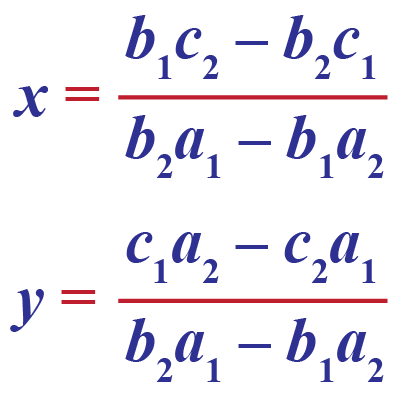### Derivation of Cross Multiplication Method

To understand this technique, consider an arbitrary pair of linear equations (that is, with any coefficients)

\begin{align}& {a_1}x + {b_1}y + {c_1} = 0\\&{a_2}x + {b_2}y + {c_2} = 0\end{align}

Let us write down the coefficients in the original pair of equations as follows, in a grid fashion

$\begin{array}{l}{a_1} & {b_1} & {c_1}\\{a_2} & {b_2} & {c_2}\end{array}$

We now will simply ignore the coefficients of $$x$$ in our grid, and cross-multiply the coefficients in the remaining two columns, and subtract them: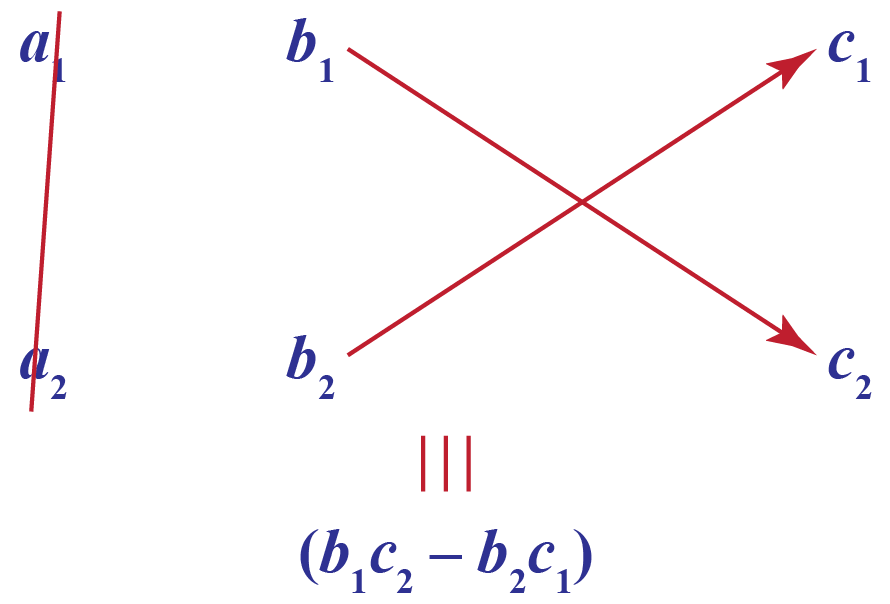Thus, the first part of our solution equality becomes

$\frac{x}{{{b_1}{c_2} - {b_2}{c_1}}}$

Next, we consider the expression below negative y, which is $${a_1}{c_2} - {a_2}{c_1}$$. To write this, we ignore the column of y coefficients, and cross-multiply the coefficients in the remaining two columns, and subtract them: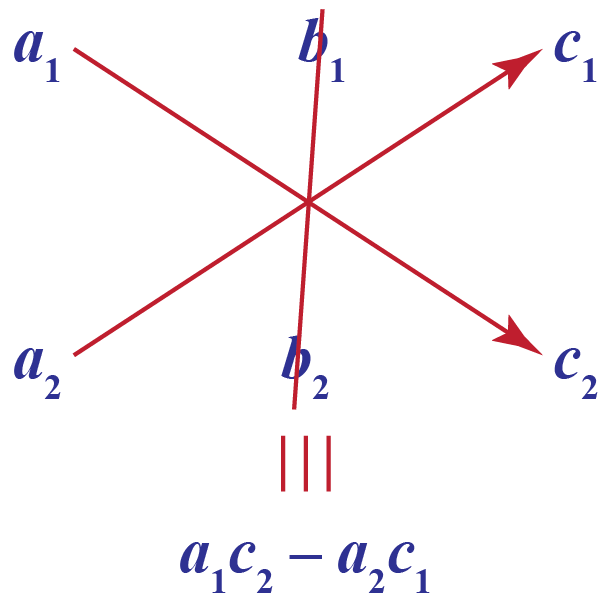Thus, the second part of our solution equality becomes,

$\frac{{ - y}}{{{a_1}{c_2} - {a_2}{c_1}}}$

Finally, we consider the expression below 1, which is $${a_1}{b_2} - {a_2}{b_1}$$. To write this, we ignore the column of constants, and cross-multiply the coefficients in the remaining two columns, and subtract them: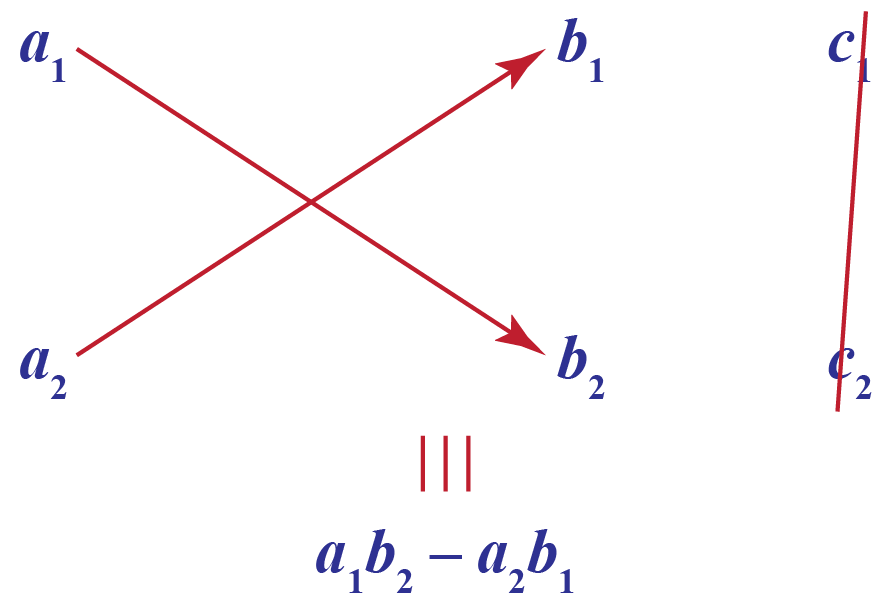Thus, the last part of our solution equality becomes

$\frac{1}{{{a_1}{b_2} - {a_2}{b_1}}}$

Combining all the three parts, our complete solution to the pair of linear equations becomes:

$\frac{x}{{{b_1}{c_2} - {b_2}{c_1}}} = \frac{{ - y}}{{{a_1}{c_2} - {a_2}{c_1}}} = \frac{1}{{{a_1}{b_2} - {a_2}{b_1}}}$

The above equation is also called the cross multiplication method formula.

That looks easy! Let us use it to solve a pair of linear equations.

## Solving Linear Equations by Cross Multiplication Method

Here is an example. Suppose that we have to solve the following pair of equations:

$\begin{array}{l}2x + 3y - 11 = 0\\3x + 2y - 9 = 0\end{array}$

Our solution equality will be of the following form, where we have to figure out the question marks:

$\frac{x}{?} = \dfrac{{ - y}}{?} = \frac{1}{?}$

To figure out the term below $$x$$, we do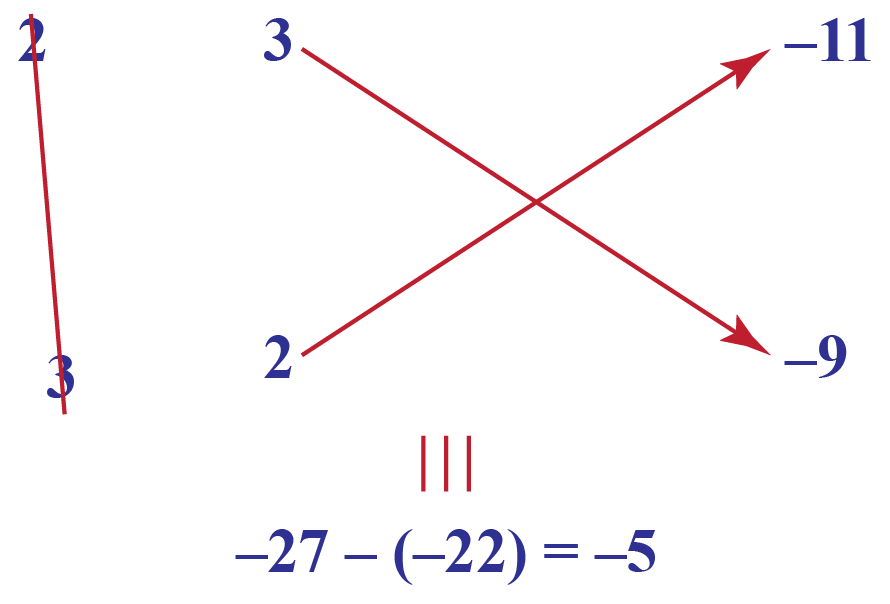Thus, the first part of the solution equality is: $$\dfrac{x}{{ - 5}}$$. Next, to figure out the term below negative $$y$$, we do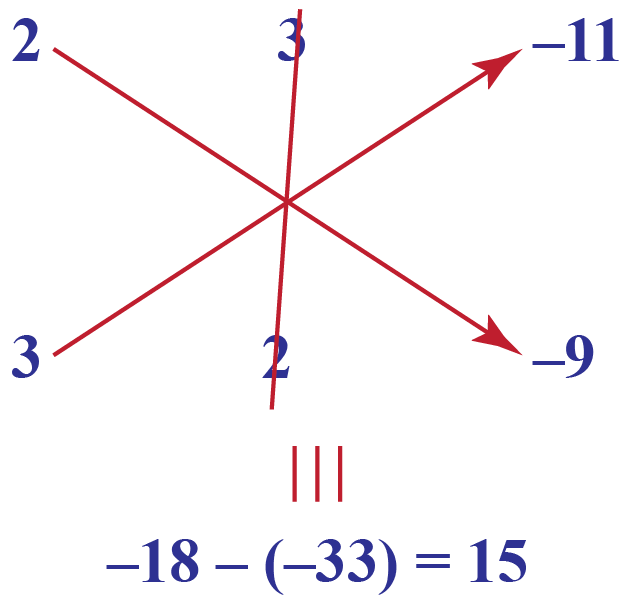Thus, the second part of the solution equality is: $$\dfrac{{ - y}}{{15}}$$. Finally, to figure out the term below 1, we do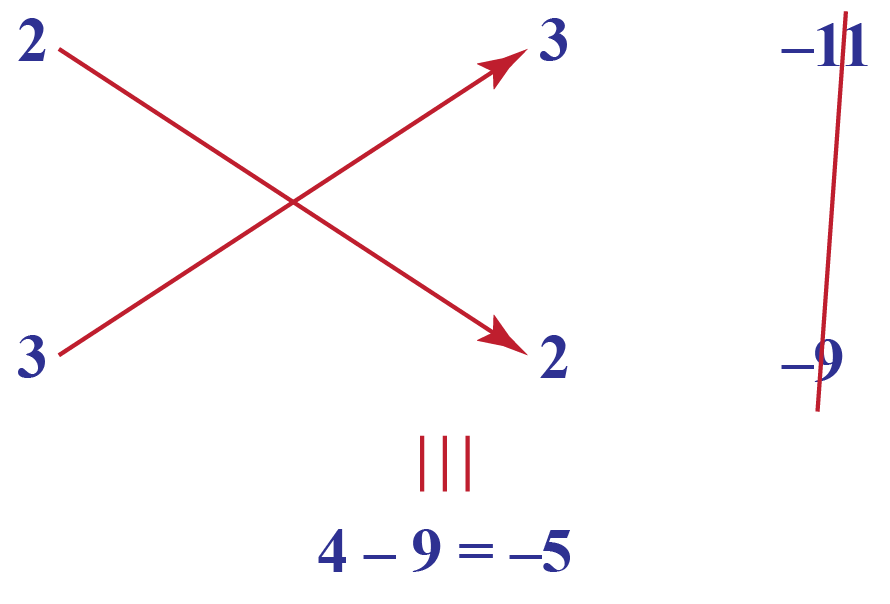Thus, the last part of the solution equality is:$$\dfrac{1}{{ - 5}}$$. Combining all the three, our complete solution is

\begin{align}&\frac{x}{{ - 5}} = \frac{{ - y}}{{15}} = \frac{1}{{ - 5}}\\ &\Rightarrow \;\;\;\left\{ \begin{gathered}\!\!\!\!\!\!\!\!\!\!\!x = \frac{{ - 5}}{{ - 5}} = 1\\y = - \left( {\frac{{15}}{{ - 5}}} \right) = 3\end{gathered} \right.\\ &\Rightarrow \;\;\;x = 1,\;y = 3\end{align}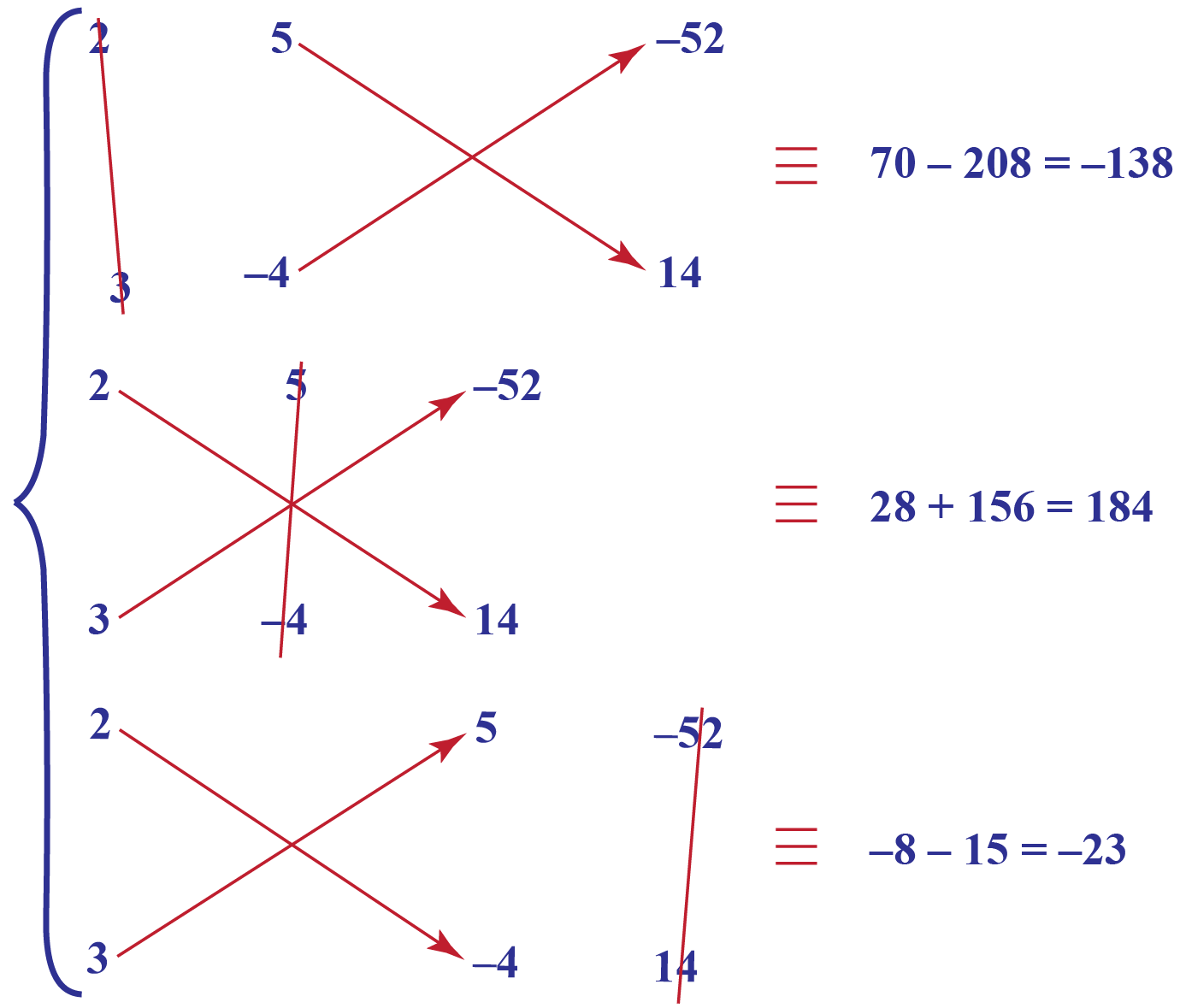Thus, the solution is

\begin{align}&\frac{x}{{ - 138}} = \frac{{ - y}}{{184}} = \frac{1}{{ - 23}}\\ &\Rightarrow \;\;\;\left\{ \begin{gathered}\!\!\!\!\!\!\!\!\!\!\!\!x = \frac{{ - 138}}{{ - 23}} = 6\\y = - \left( {\frac{{184}}{{ - 23}}} \right) = 8\end{gathered} \right.\\ &\Rightarrow \;\;\;x = 6,\;y = 8\end{align}

## Unique Solution of Linear Equations in Two Variables

On solving the linear equations in two variables by cross multiplication method do we get a unique solution?

Consider the following pair of linear equations

$\begin{array}{l}{a_1}x + {b_1}y + {c_1} = 0\\{a_2}x + {b_2}y + {c_2} = 0\end{array}$

Suppose that the cross-multiplication technique is applied to solve it. When will the pair turn out to

1. have a unique solution
2. be inconsistent
3. have infinitely many solutions

Solution

1. For the pair to have a unique solution, we must have

$\frac{{{a_1}}}{{{a_2}}} \ne \frac{{{b_1}}}{{{b_2}}}$

Thus,

\begin{align}&{a_1}{b_1} \ne {a_2}{b_1}\\&\Rightarrow \;\;\;{a_1}{b_2} - {a_2}{b_1} \ne 0\end{align}

This means that when we write the solution equality by the cross-multiplication technique, as long as the term below 1 is non-zero, there will be a unique solution: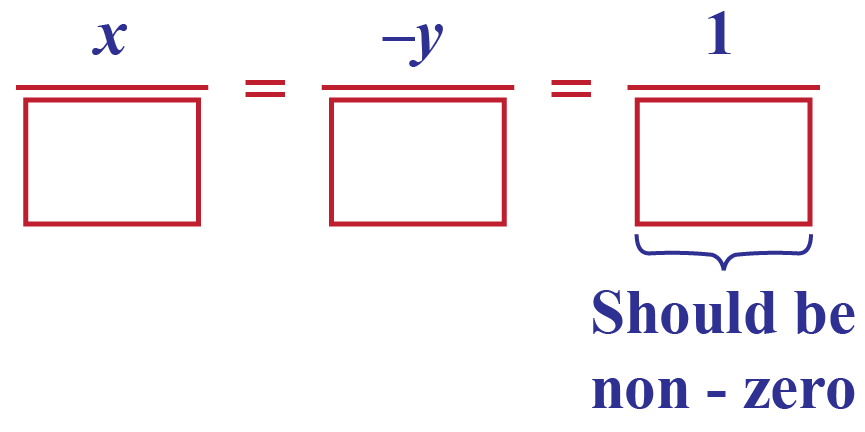2. To be inconsistent, the slopes of the two lines should be equal, but the y intercepts should be unequal. In terms of coefficients, the required conditions are

$\frac{{{a_1}}}{{{a_2}}} = \frac{{{b_1}}}{{{b_2}}} \ne \frac{{{c_1}}}{{{c_2}}}$

If you do not remember this, refer again to the discussion where we derived these conditions. Now, these conditions mean that

$\begin{array}{l}{a_1}{b_2} - {a_2}{b_1} = 0\\{b_1}{c_2} - {b_2}{c_1} \ne 0\\{a_1}{c_2} - {a_2}{c_1} \ne 0\end{array}$

This further means that when we write the solution equality by the cross-multiplication technique, if the term below 1 is zero, and either or both of the terms below x or negative y are non-zero, the pair will be inconsistent: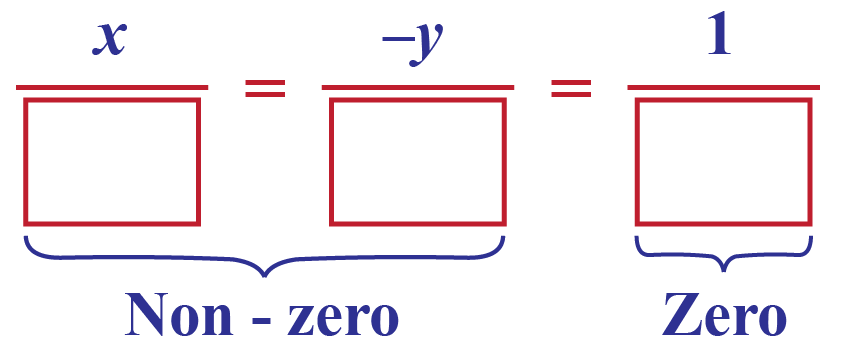3. To have infinitely many solutions, the condition on the coefficients is

$\frac{{{a_1}}}{{{a_2}}} = \frac{{{b_1}}}{{{b_2}}} = \frac{{{c_1}}}{{{c_2}}}$

Thus,

$\begin{array}{l}{a_1}{b_2} - {a_2}{b_1} = 0\\{b_1}{c_2} - {b_2}{c_1} = 0\\{a_1}{c_2} - {a_2}{c_1} = 0\end{array}$

This means that when we write the solution equality by the cross-multiplication technique, if all the three terms (below x, below negative y and below 1) are zero, the pair will have infinitely many solutions: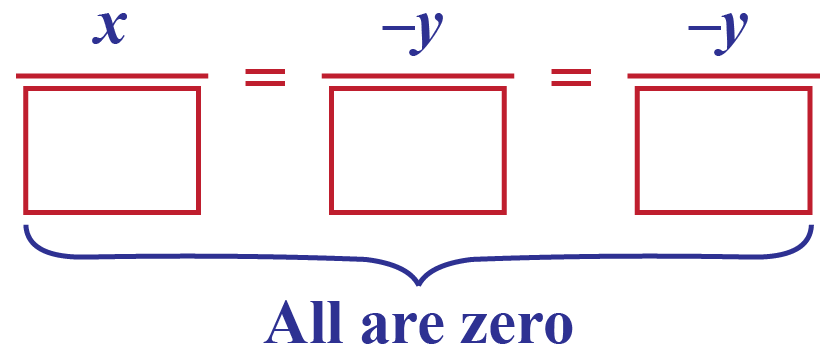Let us apply these facts to an example.

Example: Consider the following pair of linear equations:

$\begin{array}{l}2x + 7y - 1 = 0\\ - 3x + 4y + 3 = 0\end{array}$

Using the cross-multiplication technique, decide whether this pair is consistent or not.

We have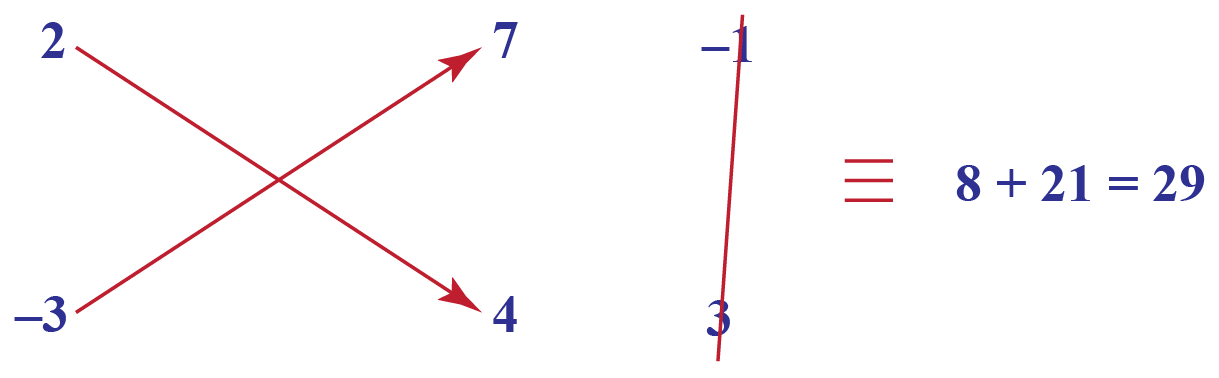Thus, in the solution equality, in the last term, we will have a non-zero denominator, which means that this pair is consistent and has a unique solution.Think Tank
1. Do we get a unique solution using the cross multiplication method?
2. Formulate a pair of equations and find the solution:

A number is greater than thrice the other number by 2. The smaller number multiplied by four times exceeds the greater by 5, what are the numbers?

## Solved Examples

 Example 1

Help Fredie to solve the following pair of linear equations by cross-multiplication

$\begin{array}{l}2x + 5y - 52 = 0\\3x - 4y + 14 = 0\end{array}$

Solution

Solution: The terms below $$x$$, negative $$y$$, and 1 are calculated below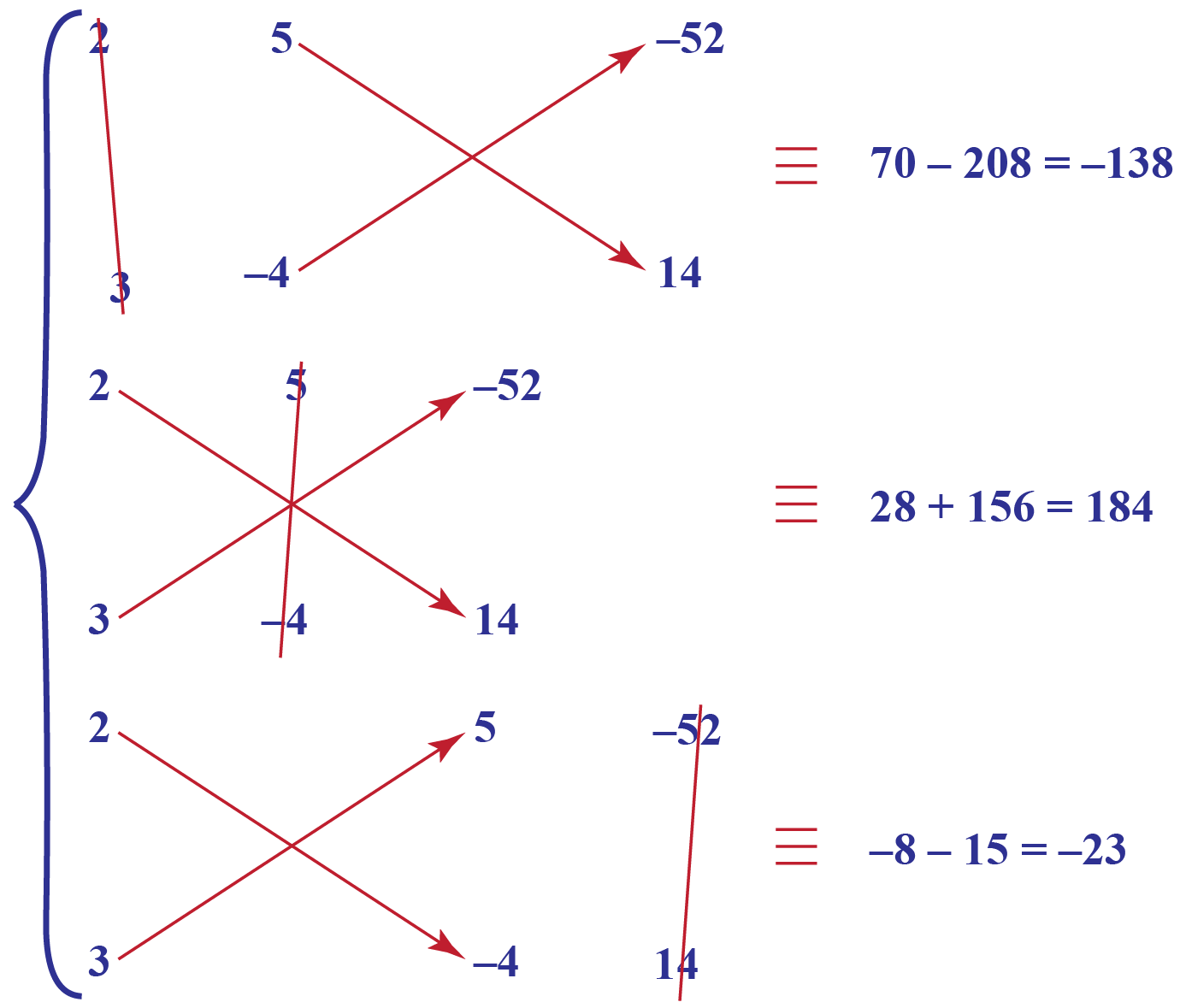Thus, the solution is

\begin{align}&\frac{x}{{ - 138}} = \frac{{ - y}}{{184}} = \frac{1}{{ - 23}}\\ &\Rightarrow \;\;\;\left\{ \begin{gathered}\!\!\!\!\!\!\!\!\!\!\!\!x = \frac{{ - 138}}{{ - 23}} = 6\\y = - \left( {\frac{{184}}{{ - 23}}} \right) = 8\end{gathered} \right.\\ &\Rightarrow \;\;\;x = 6,\;y = 8\end{align}

 $$\therefore$$ $$x = 6,\;y = 8$$
 Example 2

Joel is unable to solve the following pair of linear equations by cross-multiplication

$\begin{array}{l}3x + 4y = 32\\6x - 7y = - 11\end{array}$

Can you help him?

Solution

First, we rewrite the equations in standard form, that is, in the form $$ax + by + c = 0$$, since it is using this form that we developed our expressions to be used in the cross-multiplication technique:

$\begin{array}{l}3x + 4y - 32 = 0\\6x - 7y + 11 = 0\end{array}$

Now, we calculate the terms below x, negative y and 1 which will come in the solution equality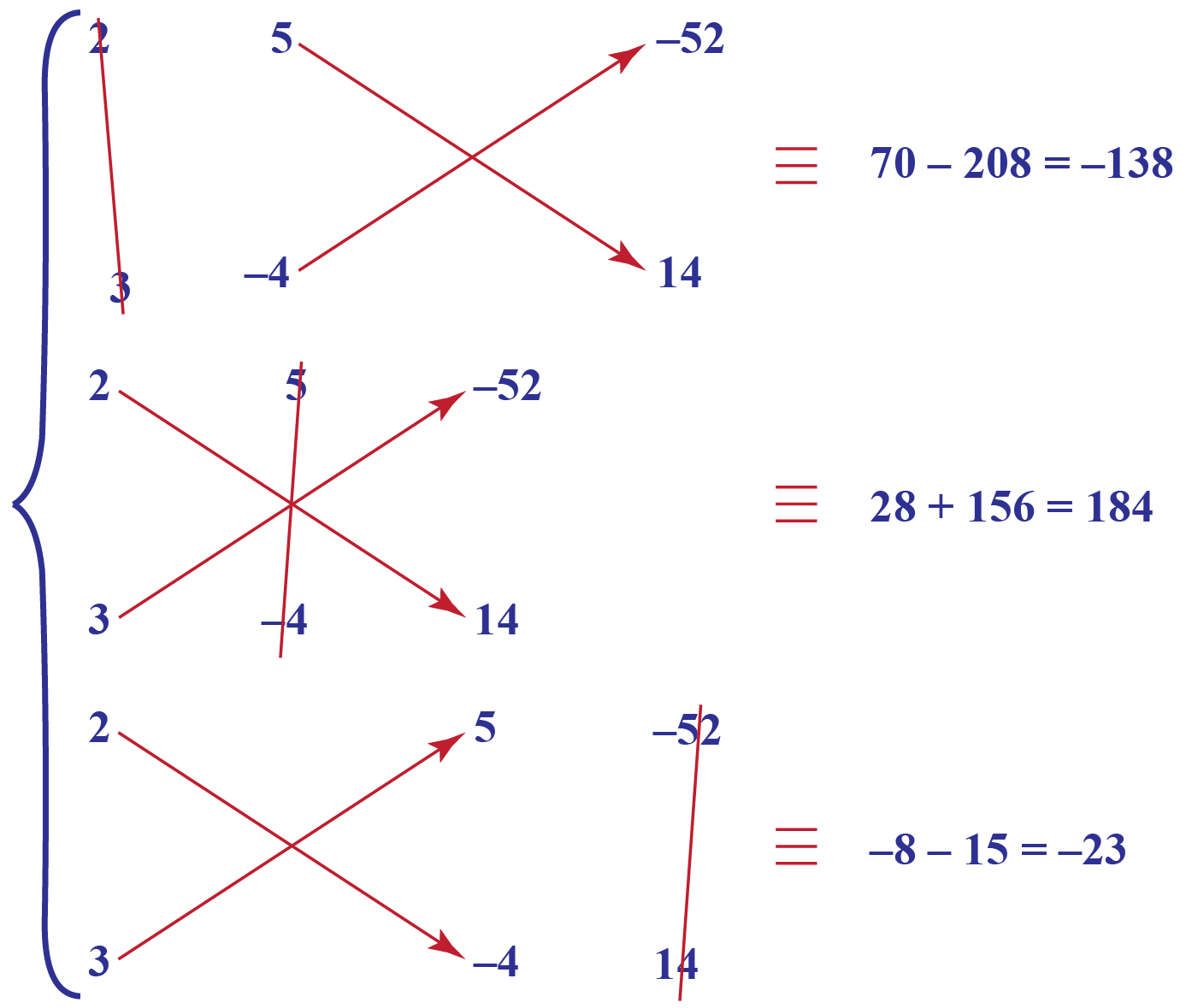Thus, the solution is

\begin{align}&\frac{x}{{ - 180}} = \frac{{ - y}}{{225}} = \frac{1}{{ - 45}}\\& \Rightarrow \;\;\;\left\{ \begin{gathered}\!\!\!\!\!\!\!\!\!\!x = \frac{{ - 180}}{{ - 45}} = 4\\y = - \left( {\frac{{225}}{{ - 45}}} \right) = 5\end{gathered} \right.\\ &\Rightarrow \;\;\;x = 4,\;y = 5\end{align}

 $$\therefore$$ $$x = 4,\;y = 5$$

## Interactive Questions

Here are a few activities for you to practice.

Select/Type your answer and click the "Check Answer" button to see the result.Challenging Questions
• Can you use cross multiplication to solve the following problems?
1. The ratio of earnings of two persons is 1:2 and the ratio of their monthly expenses is 3:7. The first person has managed to save $2000 and the second person has saved$3000, what is the expenditure of each person?
2. A mother's age is three times the sum of the age of her two daughters. After 5 years, her age will be twice the sum of the ages of her two daughters. What is the present age of the mother?

## Let's Summarize

We hope you enjoyed learning about the cross multiplication method with the examples and practice questions. Now, you will be able to easily solve problems on linear equations in two variables.

## About Cuemath

At Cuemath, our team of math experts is dedicated to making learning fun for our favorite readers, the students!

Through an interactive and engaging learning-teaching-learning approach, the teachers explore all angles of a topic.

Be it worksheets, online classes, doubt sessions, or any other form of relation, it’s the logical thinking and smart learning approach that we, at Cuemath, believe in.

## Frequently Asked Questions(FAQs)

### 1. What is the cross multiplication method?

In a cross multiplication method, the numerator of one fraction is multiplied to the denominator of another and the denominator of the first term to the numerator of another term.

### 2. How do we solve using the cross multiplication method?

Using cross multiplication the solution to the pair of linear equations in two variables becomes:

$\frac{x}{{{b_1}{c_2} - {b_2}{c_1}}} = \frac{{ - y}}{{{a_1}{c_2} - {a_2}{c_1}}} = \frac{1}{{{a_1}{b_2} - {a_2}{b_1}}}$

The above equation is also called the cross multiplication method formula.

### 3. What is the condition to get a unique solution?

\begin{align}&{a_1}{b_1} \ne {a_2}{b_1}\\&\Rightarrow \;\;\;{a_1}{b_2} - {a_2}{b_1} \ne 0\end{align}

As long as the term below 1 is non-zero, there will be a unique solution.

### 4. How do you solve cross multiplication?

Using cross multiplication the solution to the pair of linear equations in two variables becomes:

$\frac{x}{{{b_1}{c_2} - {b_2}{c_1}}} = \frac{{ - y}}{{{a_1}{c_2} - {a_2}{c_1}}} = \frac{1}{{{a_1}{b_2} - {a_2}{b_1}}}$

The above equation is also called the cross multiplication method formula.

### 5. Why does cross multiply work?

Cross multiplying fractions helps to compare them. We can use this equality to find out the value of the unknown for solving linear equations in two variables.

### 6. What do you mean by the mathematical rule of three?

The mathematical rule of three is a method that uses proportions. By using the cross multiplication method, we can find the values of unknown variables using proportion.

### 7. How many solutions are there for linear equations in two variables?

There are infinitely many solutions for linear equations in two variables.

### 8. What is a two-variable equation?

A linear equation in two variables is an equation in the form of $$ax + by + c=0$$, where $$a, b$$ & $$c$$ are real numbers and the coefficients of $$x$$ and $$y$$, i.e $$a$$ and $$b$$ are not equal to zero.

Download Pair of Linear Equations in 2 Variables Worksheets
Pair of Linear Equations in 2 Variables
grade 10 | Answers Set 1
Pair of Linear Equations in 2 Variables
grade 10 | Questions Set 2
Pair of Linear Equations in 2 Variables
grade 10 | Questions Set 1
Pair of Linear Equations in 2 Variables
grade 10 | Answers Set 2
More Important Topics
Numbers
Algebra
Geometry
Measurement
Money
Data
Trigonometry
Calculus